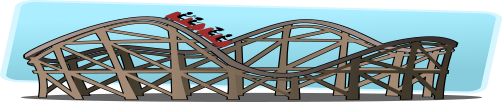# Exam-Style Questions on Approximation

## Problems on Approximation adapted from questions set in previous Mathematics exams.

### 1.

GCSE Higher

The Glacier Ski Resort has this sign showing the safety limit for each cable car:

MAXIMUM WEIGHT OF
ALL PASSENGERS
IN EACH CAR IS 500kg

Eddie and some other people are in one of the cable cars. Their total mass is 570 kg to the nearest 5 kg

Eddie gets out. He has a mass of 74 kg to the nearest kg.

Is the cable car now safe to use? You must show your working.

### 2.

GCSE Higher

Ayden is marking out the pitch for a ball game in a large field.

He measures the length of the pitch, which needs to be at least ten metres long, by pacing. Ayden knows that his stride length is 0.7 metres, rounded to the nearest tenth of a metre. Find the minimum number of strides Ayden will need to take to guarantee that the pitch is no shorter than ten metres long.

### 3.

GCSE Higher

(a) Use your calculator to evaluate the following expression to five significant figures.

$$\sqrt{8005} + \sqrt{98.5} \div 5.02$$

(b) Show how, by using approximations, your answer to part (a) is reasonable.

### 4.

GCSE Higher

(a) Work out an estimate for the value of $$\sqrt{48.3 \times 82.01}$$.

$$(3.4)^5 = 454$$ correct to 3 significant figures.

(b) Find the value of $$(0.034)^5$$ correct to 3 significant figures.

(c) Find the exact value of $$3^{–3}$$

### 5.

GCSE Higher

Minerva's Revenge, the longest rollercoaster in the Funville Theme Park, takes 95 seconds (to the nearest 5 seconds) to complete one circuit of the 2280 metre long track.(a) Mr Finklestein assumes that the track has been measured to the nearest 10m. What is the greatest possible speed of the rollercoaster train if his assumption is correct?

(b) Mr Finklestein finds out that in fact the track was measured to the nearest 5m. With this new information recalculate the greatest possible speed.

(c) Comment on the effect this new information had on the results of the calculations.

### 6.

IB Studies
$$A = \frac{(\sin(x) + y)(\cos(x) - y)}{w^2 + 4z}$$

If $$w = 12, x = 45°, y = \frac12 \text{and } z = 14$$ calculate:

(a) the exact value of $$A$$;

(b) the value of $$A$$ to two significant figures;

(c) the value of $$A$$ to three decimal places;

Rosie estimates the value of $$A$$ to be 0.0012.

(d) Calculate the percentage error in Rosie's estimate.

If you would like space on the right of the question to write out the solution try this Thinning Feature. It will collapse the text into the left half of your screen but large diagrams will remain unchanged.

The exam-style questions appearing on this site are based on those set in previous examinations (or sample assessment papers for future examinations) by the major examination boards. The wording, diagrams and figures used in these questions have been changed from the originals so that students can have fresh, relevant problem solving practice even if they have previously worked through the related exam paper.

The solutions to the questions on this website are only available to those who have a Transum Subscription.

Exam-Style Questions Main Page

Search for exam-style questions containing a particular word or phrase:

To search the entire Transum website use the search box in the grey area below.